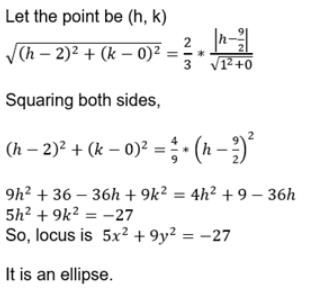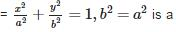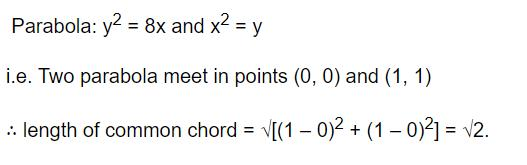Courses

# Test: Conic Sections (CBSE Level)- 2

## 25 Questions MCQ Test Mathematics For JEE | Test: Conic Sections (CBSE Level)- 2

Description
This mock test of Test: Conic Sections (CBSE Level)- 2 for JEE helps you for every JEE entrance exam. This contains 25 Multiple Choice Questions for JEE Test: Conic Sections (CBSE Level)- 2 (mcq) to study with solutions a complete question bank. The solved questions answers in this Test: Conic Sections (CBSE Level)- 2 quiz give you a good mix of easy questions and tough questions. JEE students definitely take this Test: Conic Sections (CBSE Level)- 2 exercise for a better result in the exam. You can find other Test: Conic Sections (CBSE Level)- 2 extra questions, long questions & short questions for JEE on EduRev as well by searching above.
QUESTION: 1

Solution:
QUESTION: 2

### The line y = c is a tangent to the parabola 7/2 if c is equal to

Solution:

y = x is tangent to the parabola
y=ax2+c
if a= then c=?
y′ =2ax
y’ = 2(7/2)x  =1
x = 1/7
1/7 = 2(1/7)2 + c
c = 1/7 * 2/49
c = 7/2

QUESTION: 3

### The equation 2x2+3y2−8x−18y+35 = λ Represents

Solution:

Given the equation is,
2x2+3y2−8x−18y+35=K
Or, 2{x2−4x+4} + 3{y2−6y+9}=K
Or, 2(x−2)2 + 3(y−3)2 =K.
From the above equation it is clear that if K>0 then the given equation will represent an ellipse and for K<0, no geometrical interpretation.
Also if K=0 then the given equation will be reduced to a point and the point will be (2,3).

QUESTION: 4

The locus of a variable point whose distance from the point (2, 0) is 2/3 times its distance from the line x = 9/2 is

Solution:QUESTION: 5

The axis of the parabola 9y2−16x−12y−57 = 0 is

Solution:
QUESTION: 6

A and B are two distinct points, Locus of a point P satisfying |PA| + |PB| = 2k, a constant is

Solution:
QUESTION: 7

The eccentricity of the hyperbola x2−y2 = 9 is

Solution:
QUESTION: 8

Locus of the point of intersection of the lines x = sec θ + tan θ and y = sec θ – tan θ is

Solution:
QUESTION: 9

The line y = m x + c, touches the parabola y2 = 4ax if

Solution:
QUESTION: 10

The equations x = at2, y = 4at ; t ∈ R represent

Solution:
QUESTION: 11t ∈ R represents

Solution:

P(x,y) = [(et + e-t)/2 , (et - e-t)/2]
(et + e-t)/2 = x --------------------------(1)
(e- e-t)/2 = y --------------------------(2)
2e= 2x + 2y
et = x + y
Eq (1) et + e-t = 2x
et + 1/et = 2x
(et)2 + 1 = 2x*et
(x+y)2 + 1 = 2x(x+y)
x2 + y2 + 2xy + 1 = 2x2 + 2xy
x2 + y2 + 1 = 2x2
(x2)/(1)2 - (y2)/(1)2 = 1  {which represents hyperbola equation}

QUESTION: 12

The vertex of the parabola y2 = 4a(x−a) is

Solution:
QUESTION: 13

The two parabolas x2 = 4y and y2 = 4x meet in two distinct points. One of these is the origin and the other is

Solution:
QUESTION: 14

The equation of the directrix of the parabola x2 = −4ay is

Solution:
QUESTION: 15

The eccentricity ‘e’ of a parabola is

Solution:
QUESTION: 16

The ellipseSolution:
QUESTION: 17

The equations x = a cos θ , y = b sin θ, 0 ≤ θ < 2π , a ≠ b, represent

Solution:
QUESTION: 18

The graph of the function f(x) i/x i.e. the curve y = 1/x is

Solution:
QUESTION: 19

The line y = c touches the parabola y2 = 4ax when

Solution:
QUESTION: 20

The parabolas x2 = 4y and y2 = 4x intersect

Solution:
QUESTION: 21

The lngth of the common chord of the parabolas y2 = x and x2 = y is

Solution:QUESTION: 22

The number of points on X-axis which are at a distance c units (c < 3) from (2, 3) is

Solution:

Distance of 'c' units from (2,3)
Let the no: of points be (x,0)
By distance formula
{(2−x)2+(3−0)2}=c
4−4x+x2+9=c
⇒x2−4x+13 = c:c=2,2
There are the points of c,such that when they are applied back to the equations,the number of points will become zero.

QUESTION: 23

The eccentricity of the conic 9x2 − 16y2 = 144 is

Solution:
QUESTION: 24

The eccentricity of 3x2+4y2 = 24 is

Solution:
QUESTION: 25

The angle between the tangents drawn from the origin to the circle = (x−7)2+(y+1)2 = 25 is

Solution:

Let the equation of tangent drawn from (0,0) to the circle be y=mx. Then, p = a ⇒ 7m+1/(m2+1)1/2= 5
⇒24m2 + 14m−24=0
⇒12m2 + 7m−12=0
⇒m1m2 = −12/12 =−1
∴ Required angle = π/2